# JP4094851B2 - PLL circuit - Google Patents

## Info

Publication number
JP4094851B2
JP4094851B2 JP2001553665A JP2001553665A JP4094851B2 JP 4094851 B2 JP4094851 B2 JP 4094851B2 JP 2001553665 A JP2001553665 A JP 2001553665A JP 2001553665 A JP2001553665 A JP 2001553665A JP 4094851 B2 JP4094851 B2 JP 4094851B2
Authority
JP
Japan
Prior art keywords
gain
vco
circuit
signal
based
Prior art date
Legal status (The legal status is an assumption and is not a legal conclusion. Google has not performed a legal analysis and makes no representation as to the accuracy of the status listed.)
Expired - Fee Related
Application number
JP2001553665A
Other languages
Japanese (ja)
Inventor

Original Assignee

Priority date (The priority date is an assumption and is not a legal conclusion. Google has not performed a legal analysis and makes no representation as to the accuracy of the date listed.)
Filing date
Publication date
Application filed by 富士通株式会社 filed Critical 富士通株式会社
Priority to PCT/JP2000/000170 priority Critical patent/WO2001054283A1/en
Application granted granted Critical
Publication of JP4094851B2 publication Critical patent/JP4094851B2/en
Anticipated expiration legal-status Critical
Application status is Expired - Fee Related legal-status Critical

## Images

•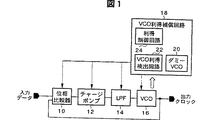•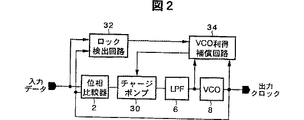•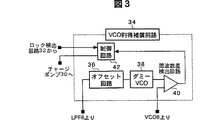•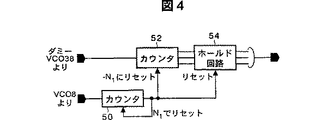••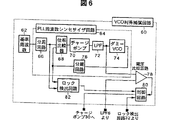•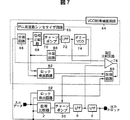•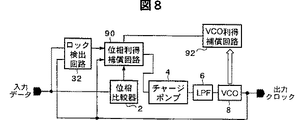•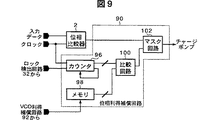•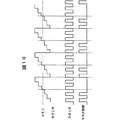•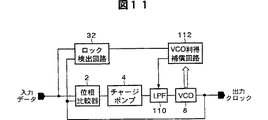•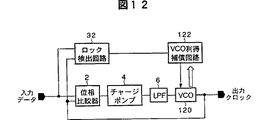•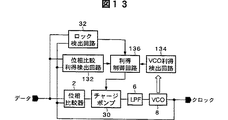•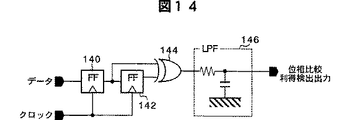•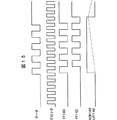•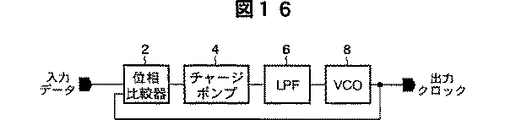•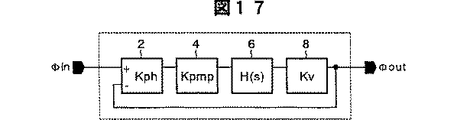••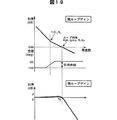•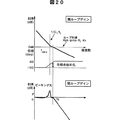•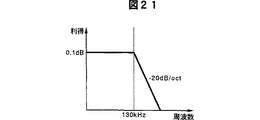•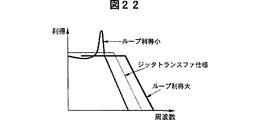## Classifications

• HELECTRICITY
• H03BASIC ELECTRONIC CIRCUITRY
• H03LAUTOMATIC CONTROL, STARTING, SYNCHRONISATION, OR STABILISATION OF GENERATORS OF ELECTRONIC OSCILLATIONS OR PULSES
• H03L7/00Automatic control of frequency or phase; Synchronisation
• H03L7/06Automatic control of frequency or phase; Synchronisation using a reference signal applied to a frequency- or phase-locked loop
• H03L7/08Details of the phase-locked loop
• H03L7/0805Details of the phase-locked loop the loop being adapted to provide an additional control signal for use outside the loop
• HELECTRICITY
• H03BASIC ELECTRONIC CIRCUITRY
• H03LAUTOMATIC CONTROL, STARTING, SYNCHRONISATION, OR STABILISATION OF GENERATORS OF ELECTRONIC OSCILLATIONS OR PULSES
• H03L7/00Automatic control of frequency or phase; Synchronisation
• H03L7/06Automatic control of frequency or phase; Synchronisation using a reference signal applied to a frequency- or phase-locked loop
• H03L7/07Automatic control of frequency or phase; Synchronisation using a reference signal applied to a frequency- or phase-locked loop using several loops, e.g. for redundant clock signal generation
• HELECTRICITY
• H03BASIC ELECTRONIC CIRCUITRY
• H03LAUTOMATIC CONTROL, STARTING, SYNCHRONISATION, OR STABILISATION OF GENERATORS OF ELECTRONIC OSCILLATIONS OR PULSES
• H03L7/00Automatic control of frequency or phase; Synchronisation
• H03L7/06Automatic control of frequency or phase; Synchronisation using a reference signal applied to a frequency- or phase-locked loop
• H03L7/08Details of the phase-locked loop
• HELECTRICITY
• H03BASIC ELECTRONIC CIRCUITRY
• H03LAUTOMATIC CONTROL, STARTING, SYNCHRONISATION, OR STABILISATION OF GENERATORS OF ELECTRONIC OSCILLATIONS OR PULSES
• H03L7/00Automatic control of frequency or phase; Synchronisation
• H03L7/06Automatic control of frequency or phase; Synchronisation using a reference signal applied to a frequency- or phase-locked loop
• H03L7/08Details of the phase-locked loop
• H03L7/085Details of the phase-locked loop concerning mainly the frequency- or phase-detection arrangement including the filtering or amplification of its output signal
• H03L7/089Details of the phase-locked loop concerning mainly the frequency- or phase-detection arrangement including the filtering or amplification of its output signal the phase or frequency detector generating up-down pulses
• H03L7/0891Details of the phase-locked loop concerning mainly the frequency- or phase-detection arrangement including the filtering or amplification of its output signal the phase or frequency detector generating up-down pulses the up-down pulses controlling source and sink current generators, e.g. a charge pump
• H03L7/0895Details of the current generators
• H03L7/0898Details of the current generators the source or sink current values being variable
• HELECTRICITY
• H03BASIC ELECTRONIC CIRCUITRY
• H03LAUTOMATIC CONTROL, STARTING, SYNCHRONISATION, OR STABILISATION OF GENERATORS OF ELECTRONIC OSCILLATIONS OR PULSES
• H03L7/00Automatic control of frequency or phase; Synchronisation
• H03L7/06Automatic control of frequency or phase; Synchronisation using a reference signal applied to a frequency- or phase-locked loop
• H03L7/08Details of the phase-locked loop
• H03L7/085Details of the phase-locked loop concerning mainly the frequency- or phase-detection arrangement including the filtering or amplification of its output signal
• H03L7/093Details of the phase-locked loop concerning mainly the frequency- or phase-detection arrangement including the filtering or amplification of its output signal using special filtering or amplification characteristics in the loop
• HELECTRICITY
• H03BASIC ELECTRONIC CIRCUITRY
• H03LAUTOMATIC CONTROL, STARTING, SYNCHRONISATION, OR STABILISATION OF GENERATORS OF ELECTRONIC OSCILLATIONS OR PULSES
• H03L7/00Automatic control of frequency or phase; Synchronisation
• H03L7/06Automatic control of frequency or phase; Synchronisation using a reference signal applied to a frequency- or phase-locked loop
• H03L7/08Details of the phase-locked loop
• H03L7/085Details of the phase-locked loop concerning mainly the frequency- or phase-detection arrangement including the filtering or amplification of its output signal
• H03L7/095Details of the phase-locked loop concerning mainly the frequency- or phase-detection arrangement including the filtering or amplification of its output signal using a lock detector

## Description

The present invention relates to a PLL (Phased Lock Loop) circuit, and more particularly to control of the gain of a PLL circuit.

The PLL circuit is a circuit that performs feedback control so that the phase difference between the input signal and the output signal of the oscillator is kept constant. The PLL circuit is used for clock extraction or the like in optical communication or the like.

FIG. 16 is a configuration diagram of a conventional PLL circuit. As shown in FIG. 16, the PLL circuit includes a phase comparator 2, a charge pump 4, an LPF 6, and a voltage controlled oscillation circuit (VCO) 8. The phase comparator 2 compares the phase of the input clock such as NRZ data and the output clock of the VCO 8 and outputs a phase difference signal indicating the phase difference (for example, indicating the phase difference by the pulse width) to the charge pump 4. . The charge pump 4 converts the phase difference signal into a current and outputs an output current. The LPF 6 smoothes the output current. The VCO 8 oscillates at an oscillation frequency according to the output voltage of the LPF 6 and outputs a clock. An output clock of the VCO 8 is input to the phase comparator 2 and fed back to the VCO 8 to obtain a clock synchronized with the input data.

FIG. 17 is a block diagram of FIG. As shown in FIG. 17, the closed loop gain of the feedback loop of the PLL circuit is expressed by Expression (1). Further, the open loop gain is expressed by Expression (2).

Open loop gain = Kph, Ipmp, F (s), Kv / s (1)
Closed loop gain = Φout / Φin
= Kph · Impmp · F (s) · Kv / {s + Kph · ImpmpF (s) Kv}
... (2)
Where Kph is a phase comparator gain, for example, pulse width / phase difference (s / rad), Ipmp is a charge pump current amplitude value (A), and F (s) is an LPF transfer function, for example, voltage / The current value (Ω) and Kv are VCO gains and are frequency / voltage (Hz / V).

FIG. 18 is a diagram illustrating a complete second-order loop filter. F (s) of the complete quadratic loop shown in FIG.

F (s) = R1 (1 + 1 / sC1 · R1) (3)
The open loop gain and the closed loop gain when a complete secondary loop filter is used in the PLL circuit are expressed by equations (4) and (5) when equation (3) is substituted into equations (1) and (2), respectively. .

Open loop gain = (Kph · Ipmp · KvR1 / s) · (1 + 1 / sC1R1)
... (4)
Closed loop gain = {Kph · Ipmp · Kv · (1 + sC1R1)}
/ {S 2 C1 + Kph · Ipmp · Kv · (1 + sC1 · R1)}
... (5)
19 and 20 are diagrams showing the frequency characteristics of the PLL circuit expressed by the equations (4) and (5). 19 and 20, the upper stage represents the gain characteristics and phase characteristics of the open loop gain, and the lower stage represents the closed loop gain. FIG. 19 shows a case where the loop gain is large, and FIG. 20 shows a case where the loop gain is small.

The open loop gain decreases from the low frequency side to 1 / R1C1 at 12 dB / oct, and from 1 / R1C1 to 6 dB / oct. The cutoff frequency of the closed loop gain is a frequency at which the open loop gain becomes approximately 0 dB, and is proportional to the loop gain represented by the equation (6).

Closed loop gain cutoff frequency ≒ Loop gain = Kph, Ipmp, Kv, R1
... (6)
In Equation (6), 1 << R1C1.

Therefore, the cutoff frequency of the closed loop gain increases in proportion to the gains of the phase comparator, charge pump, and VCO.

As shown in FIG. 19, when the loop gain is large, the phase margin is large (90 deg) and the cutoff frequency is large. Conversely, as shown in FIG. 20, when the loop gain is small, the phase margin is small (about 45 degrees), so that peaking increases and the cut-off frequency decreases. When the cut-off frequency is increased, the high-frequency phase fluctuation is not cut and the jitter is increased. On the other hand, when the phase margin decreases and the peaking increases, the phase fluctuation, that is, jitter increases. In general, the closed loop gain characteristic is defined by jitter transfer. The closed loop gain of the PLL circuit used for clock extraction in optical communication or the like is defined by the STM-1 jitter transfer specification of ITU-T recommendation G958.

FIG. 21 is a diagram showing the specifications of the jitter transfer of STM-1 of ITI-T recommendation G958. As shown in FIG. 21, in this specification, the cutoff frequency is 130 kHz or less and the peaking is 0.1 dB or less.

FIG. 22 is a diagram illustrating the PLL frequency characteristics when the loop gain varies. If the loop gain is too small, the peaking specification cannot be satisfied as shown in FIG. On the other hand, if the loop gain is too large, the cutoff specification cannot be satisfied as shown in FIG. Therefore, in order to satisfy the jitter transfer, it is necessary to design the loop gain of the PLL circuit to be between the lower limit value determined from the peaking specification and the upper limit value determined from the cutoff frequency specification.

However, when a VCO that operates at a high frequency is designed, the VCO gain KV varies greatly depending on the process, temperature, and power supply suppression, so that the loop gain varies greatly and it is difficult to satisfy the jitter transfer characteristics.

In response to this problem, Japanese Patent Application No. 8-310804 discloses that the VCO gain is detected from the frequency convergence characteristic of the PLL circuit and the loop gain of the PLL circuit is compensated. In this example, the VCO gain Kv is detected from the time change of the VCO control voltage when the PLL circuit converges. The time change of the VCO control voltage is sampled by an A / D converter and calculated by a DSP to detect and compensate for the VCO gain. However, in order to detect the time change of the VCO control voltage, it is necessary to once unlock the PLL circuit, so that the change in the VCO gain due to the change in temperature and power supply voltage during continuous operation like a communication device or the like. There was a problem that could not be handled. Further, since an A / D conversion circuit and a DSP are required, the circuit is complicated.

An object of the present invention is to provide a PLL circuit that measures the VCO gain during continuous operation with a simple circuit configuration and makes the gain of the PLL circuit constant.

According to an aspect of the present invention, a PLL circuit having a gain control function, the first phase comparator outputting a first phase difference signal indicating a phase difference between the first input signal and the second input signal, A first loop filter that smoothes a signal based on one phase difference signal and outputs a first control voltage, and a VCO (voltage controlled oscillation circuit) that oscillates at a frequency based on the first control voltage and outputs a first clock ), A dummy VCO having the same characteristics as the VCO that oscillates at a frequency based on the second control voltage and outputs a second clock, the frequency difference between the first and second clocks, and the first and second control voltages And a gain control circuit for controlling the loop gain to be constant based on the gain of the VCO detected by the VCO gain detection circuit. and, the 1 control voltage by adding an offset voltage to, comprises an offset circuit for outputting the dummy VCO the added voltage as said second control voltage, said second input signal is a signal based on the first clock A PLL circuit having a gain control function is provided.

The gain of the VCO varies due to variations in the operating environment. The VCO gain detection circuit uses a dummy VCO having the same characteristics as the VCO, and uses a first control frequency V1 applied to the VCO when the PLL circuit is locked, a first control voltage V1 applied to the VCO, and a second control applied to the dummy VCO. According to the second oscillation frequency f1 of the voltage V2 and the dummy VCO, the gain of the VCO is detected by (Δf2−Δf1) / Δ (V2−V1). Control is performed so that the loop gain of the PLL circuit becomes constant according to the gain of the VCO. Thereby, even if the gain of the VCO fluctuates, the PLL circuit satisfies the jitter transfer specifications.

According to the present invention, using a dummy VCO having the same characteristics as the VCO, according to the oscillation frequency of the VCO when the PLL circuit is locked, the control voltage applied to the VCO, the control voltage applied to the dummy VCO, and the oscillation frequency of the dummy VCO, The gain of the VCO is detected, and the loop gain of the PLL circuit is controlled to be constant according to the gain of the VCO. Thereby, even if the gain of the VCO fluctuates, the PLL circuit satisfies the jitter transfer specifications.

According to the present invention, the edge rate of the digital data is detected and controlled so that the loop gain of the PLL circuit becomes constant according to the gain of the phase comparator indicated by the edge rate. Therefore, even if the gain of the phase comparator varies due to the variation of the edge rate, the PLL circuit satisfies the jitter transfer specifications.

Before describing the best mode for carrying out the invention, the principle of the present invention will be described. FIG. 1 shows the principle of the present invention. As shown in FIG. 1, the PLL circuit includes a phase comparator 10, a charge pump 12, an LPF 14, a VCO 16, and a VCO gain compensation circuit 18. The VCO gain compensation circuit 18 includes a dummy VCO 20, a VCO gain detection circuit 22, and a gain control circuit 24.

The phase comparator 10 receives input data such as NRZ data such as optical communication and an output clock of the VCO 16. The phase comparator 10 detects the phase difference between the input data and the output clock of the VCO 16 and outputs a phase difference signal indicating the phase difference. This phase difference signal is, for example, a signal indicating the phase difference with a pulse width. The charge pump 12 converts the phase difference signal into a current and outputs it to the LPF 14. The LPF 14 converts the output current of the charge pump 12 into a voltage V and outputs it to the VCO 16. The VCO 16 oscillates at a frequency according to the voltage V and outputs a clock.

The gain of the VCO 16 varies depending on the operating environment. The VCO gain detection circuit 22 detects the gain of the VCO 16 using the dummy VCO 20 having the same characteristics as the VCO 16. For example, when the PLL circuit is locked, the output frequency of the VCO 16 during operation, the voltage (V + ΔV) obtained by adding the offset voltage ΔV to the voltage V, and the gain of the VCO 16 can be detected from the output frequency of the dummy VCO 20 and the offset voltage ΔV. it can. The gain control circuit 24 controls the gain of the phase comparator 10, the charge pump 12, the LPF 14, and the VCO 16 so that the loop gain of the PLL circuit becomes constant from the gain of the VCO 16. Thereby, even if the gain of the VCO 16 varies, the gain of the PLL circuit can be made constant while the PLL circuit is operating.

First Embodiment FIG. 2 is a block diagram of a PLL circuit according to a first embodiment of the present invention. Components that are substantially the same as those in FIG. 16 are given the same reference numerals. The PLL circuit shown in FIG. 2 is used for an optical communication clock extraction circuit or the like. This PLL circuit includes a phase comparator 2, a charge pump 30, an LPF 6, a VCO 8, a lock detection circuit 32, and a VCO gain compensation circuit 34.

The phase comparator 2 detects the phase difference between the input data and the output clock of the VCO 8 and outputs a phase difference signal composed of a pulse signal indicating the phase difference in pulse width and an UP / DOWN signal indicating phase delay / advance. . The charge pump 30 includes a positive current source circuit and a negative current source circuit whose output amplitude current value Ipmp is controlled by the VCO gain compensation circuit 32, and a first switch and a negative constant current that connect between the positive constant current source circuit and the LPF 6. A second switch for connecting between the source circuit and the LPF 6 is provided. In response to the UP signal / DOWN signal, the first / second switch is turned on for the pulse width of the pulse signal, and the output current Ipmp or -Ipmp is output to the LPF 6.

The LPF 6 smoothes the output current of the charge pump 30 and outputs a smoothed voltage V. For example, the LPF 6 is a complete second-order filter shown in FIG. The VCO 8 oscillates at an oscillation frequency according to the output voltage V of the LPF 6 and outputs an output clock, for example, a ring oscillator. The lock detection circuit 32 detects that the phase of the output clock of the VCO 8 matches the phase of the input data and locks the PLL circuit, and outputs a lock detection signal to the VCO gain compensation circuit 34.

The VCO gain compensation circuit 34 outputs the output frequency f1 of the VCO 8 when the PLL circuit is locked and the voltage (V + ΔV) obtained by adding the offset voltage ΔV to the output voltage V of the LPF 6 at that time to the dummy VCO having the same gain as the VCO 8. From the output frequency f2 when applied, the gain Kv of the VCO 8 represented by the equation (7) is measured.

Kv = change in output frequency of VCO8 / change in voltage = (f2-f1) / ΔV (7)
In equation (7), f2 is preferably the output frequency at the voltage (V + ΔV) of the VCO 8, but the output frequency of the VCO 8 cannot be changed while the VCO 8 is locked. Therefore, the output frequency f2 is measured using a dummy VCO having the same characteristics as the VCO 8. At this time, the gain of the dummy VCO also fluctuates due to a change in the operating environment. However, since the operating environment is the same as that of the VCO 8, the fluctuating gain is also estimated to be the same as the VCO 8. Therefore, the gain Kv of the VCO 8 is detected.

The gain Ipmp and gain Kv of the charge pump 30 are compensated for by compensating for fluctuations in the gain Kv of the VCO 8 due to changes in the operating environment such as temperature change and power supply voltage, so that the PLL circuit matches the loop gain satisfying the jitter transfer specifications. The gain of the charge pump 30 (amplitude current of the constant current source circuit) is controlled so that the product Ipmp × Kv of

FIG. 3 is a block diagram of the VCO gain compensation circuit 34 in FIG. As shown in FIG. 3, the VCO gain control circuit 34 includes an offset circuit 36, a dummy VCO 38, a frequency difference detection circuit 40, and a control circuit 42. The offset circuit 36 adds the offset voltage ΔV to the output voltage V of the LPF 6 and outputs a voltage (V + ΔV). Note that the offset voltage ΔV is a value with the best measurement accuracy of the gain Kv expressed by the equation (7). The dummy VCO 38 oscillates in accordance with the output voltage (V + ΔV) of the offset circuit 36 and outputs an output clock having an output frequency f2, and has the same characteristics as the VCO 8. The frequency difference detection circuit 40 detects the difference between the output frequency f2 of the dummy VCO 38 and the output frequency f1 of the VCO 8.

FIG. 4 is a configuration diagram of the frequency difference detection circuit 40 in FIG. As shown in FIG. 4, the frequency difference detection circuit 40 includes counters 50 and 52 and a hold circuit 54. The counter 50 counts the output clock of the VCO 8 and outputs a reset signal when it reaches a fixed value N1. The reset signal resets the counter 50 to 0 and the counter 52 to -N1. The counter 52 counts the output clock of the dummy VCO 38 and is reset to -N1 by the reset signal. The hold circuit 54 holds the counter value N2 of the counter 52 immediately before being reset until the next reset, and is, for example, a flip-flop.

Since the output frequency of the VCO 8 is f1, the time until the counter 50 counts N1 is N1 / f1. The counter 52 counts N1 / f1 ÷ (1 / f2) = (N1 × f2 / f1) times during N1 / f1. Therefore, the count value N2 of the counter 52 immediately before being reset to −N1 is (N1 × f2 / f1−N1) = ((f2−f1) / f1) × N1. Therefore, N2 = ((f2−f1) / f1) × N1 is held in the hold circuit 54. When the PLL circuit is locked, since the frequency f1 is the frequency of the bit rate of the input data and is constant, the value of N2 is proportional to the frequency difference (f2−f1).

When the lock detection signal indicates that the PLL circuit is locked, the control circuit 42 in FIG. 3 uses the frequency difference (f2-f1) from N2 = ((f2-f1) / f1) × N1 output from the frequency difference detection circuit 40. ). The gain Kv = (f2−f1) / ΔV of the VCO 8 shown in the equation (7) is calculated. The gain Ipmp of the charge pump 30 = the target value C ÷ the measured gain Kv is calculated.

The operation of the PLL circuit of FIG. 2 will be described below.

(A) Phase synchronization The phase comparator 2 receives NRZ data, which is input data for optical communications, and the output clock of the VCO 8. The phase comparator 2 compares the phase of the NRZ data with the output clock of the VCO 8 and includes a pulse signal indicating the phase difference in pulse width and an UP / DOWN signal indicating the advance / lag of the phase of the output clock with respect to the NRZ data. The phase difference signal is output to the charge pump 30. When the lock detection signal indicates that the PLL circuit is not locked, the VCO gain compensation circuit 34 determines that the target value C ÷ (the gain Kv of the VCO 8 predicted in a standard operating environment) is the gain of the charge pump 30. Control to be The charge pump 30 outputs an output current having a current amplitude Ipmp controlled by the VCO gain compensation circuit 34 to the LPF 6.

The LPF 6 smoothes the output current of the charge pump 30 by, for example, a complete secondary filter and outputs a smoothed voltage V. The VCO 8 oscillates at an oscillation frequency according to the output voltage V of the LPF 6 by a ring oscillator, for example, and outputs an output clock. The output clock of the VCO 8 is fed back to the phase comparator 2. The frequency of the VCO 8 is controlled by the phase comparator 2, the charge pump 30, and the LPF 6 so as to match the phase of the NRZ data. As a result, the PLL circuit is locked.

(B) Lock detection The lock detection circuit 32 compares the phase of the NRZ data and the VCO 8 and detects whether or not the phases match, thereby indicating whether or not the PLL circuit is locked. Is output to the VCO gain compensation circuit 34.

(C) VCO 8 Gain Detection The offset circuit 36 in the VCO gain compensation circuit 34 adds the offset voltage ΔV to the output voltage V of the LPF 6 and outputs the voltage (V + ΔV) to the dummy VCO 38. The counter 50 in the frequency difference detection circuit 40 counts the output clock of the VCO 8 and outputs a reset signal when it reaches a fixed value N1. The reset signal resets the counter 50 to 0 and the counter 52 to -N1. The counter 52 counts the output clock of the dummy VCO 38 and is reset to -N1 by the reset signal. The hold circuit 54 holds the counter value N2 = ((f2−f1) / f1) × N1 of the counter 52 immediately before resetting until the next reset. The dummy VCO 38 outputs an output clock having the oscillation frequency f2 in accordance with the voltage (V + ΔV). When the lock detection signal indicates that the PLL circuit is locked, the control circuit 42 in FIG. 3 uses the frequency difference (f2-f1) from N2 = ((f2-f1) / f1) × N1 output from the frequency difference detection circuit 40. ). The gain Kv = (f2−f1) / ΔV of the VCO 8 is calculated.

(D) Gain Control of Charge Pump 30 The control circuit 42 calculates the gain Ipmp = (the target value C ÷ the detected gain Kv) of the charge pump 30. The constant current source circuit of the charge pump 30 is controlled so as to obtain the calculated gain Ipmp. The charge pump 30 outputs an output current having a current amplitude of gain Ipmp to the LPF 6 under the control of the control circuit 42. As a result, even if the gain of the VCO 8 changes due to temperature changes or fluctuations in the power supply voltage, the loop gain of the PLL circuit becomes constant, and the PLL circuit satisfies the jitter transfer specifications. Moreover, the loop gain of the PLL circuit can be controlled to be constant without releasing the lock during the operation of the PLL circuit.

Second Embodiment FIG. 5 is a block diagram of a PLL circuit according to a second embodiment of the present invention. Components substantially the same as those in FIG. 2 are denoted by the same reference numerals. As shown in FIG. 5, the PLL circuit includes a phase comparator 2, a charge pump 30, an LPF 6, a VCO 8, a lock detection circuit 32, and a VCO gain compensation circuit 60.

The VCO gain compensation circuit 60 has a PLL frequency synthesizer circuit including a dummy VCO having the same characteristics as the VCO 8. The output voltage V1 of the LPF 6 when the PLL circuit is locked to the known frequency f1 of the bit rate of the NRZ data, the output voltage V2 of the LPF when the PLL frequency synthesizer circuit is locked to the known frequency f2, and the frequency difference ( The gain Kv of the VCO 8 is detected by f2-f1), and the gain of the charge pump 30 is controlled so that the loop gain of the PLL circuit becomes constant.

FIG. 6 is a configuration diagram of the VCO gain compensation circuit 60 in FIG. As shown in FIG. 6, the VCO gain compensation circuit 60 includes a reference frequency oscillation circuit 62, a dummy frequency synthesizer circuit 64, a voltage comparison circuit 78, and a control circuit 80. The reference frequency oscillation circuit 62 outputs a clock signal having a reference frequency f3. The PLL frequency synthesizer circuit 64 includes a frequency divider circuit 66, a phase comparator 68, a charge pump 70, an LPF 72, a dummy VCO 74, and a frequency divider circuit 76.

The frequency dividing circuit 66 divides the reference clock having the reference frequency f3 by the frequency dividing ratio 1 / N1 and outputs the clock having the frequency f3 / N1. The phase comparator 68 detects the phase difference between the output clock of the frequency divider circuit 66 and the output clock of the frequency divider circuit 76, and a pulse signal indicating the phase difference in pulse width and an UP / DOWN signal indicating phase delay / advance. A phase difference signal consisting of The charge pump 70 converts the pulse signal into a current and outputs it to the LPF 72. The LPF 72 smoothes the output current of the charge pump 70 and outputs a smoothed voltage V2. The dummy VCO 74 oscillates at an oscillation frequency f2 according to the output voltage V2 of the LPF 72, and outputs an output clock. The frequency dividing circuit 76 divides the output clock having the frequency f2 by the frequency dividing ratio 1 / N2. When the PLL frequency synthesizer circuit 64 is locked, f2 = (f3 × N2) / N1.

The voltage comparison circuit 78 calculates a differential voltage ΔV = (V2−V1) between the output voltage V1 of the LPF 6 and the output voltage V2 of the LPF 72, and is, for example, an operational amplifier. When the lock detection signals of the lock detection circuits 32 and 82 indicate that the PLL circuit and the PLL frequency synthesizer circuit 64 are locked, the control circuit 80 knows the known frequency f2 of the dummy VCO 74 and the known frequency f1 of the bit rate of the input data. The gain Kv of the VCO 8 shown in the equation (8) is calculated from the differential voltage ΔV.

Kv = (f2-f1) / ΔV (8)
The control circuit 80 calculates the gain Ipmp = (the target value C ÷ the gain K V detected by the equation (8)) of the charge pump 30. The constant current source circuit of the charge pump 30 is controlled so as to obtain the calculated gain Ipmp. The lock detection circuit 82 compares the output clocks of the frequency dividing circuits 68 and 76, detects whether or not the PLL frequency synthesizer circuit 64 is locked, and outputs a lock detection signal.

The operation of the PLL circuit in FIG. 5 will be described below.

(A) Phase synchronization is achieved in the same manner as (a) of the first embodiment.

(B) Lock detection The lock detection circuit 32 compares the phase of the NRZ data with the VCO 8 to detect whether the phases match, and outputs a lock detection signal to the VCO gain compensation circuit 34. On the other hand, the lock detection circuit 82 compares the output clocks of the frequency dividing circuits 68 and 76, detects whether the PLL frequency synthesizer circuit 64 is locked, and outputs a lock detection signal.

(C) VCO 8 Gain Detection The reference frequency oscillation circuit 62 in the VCO gain compensation circuit 60 outputs a clock signal having a frequency f2. The frequency dividing circuit 66 divides the reference clock having the reference frequency f3 by the frequency dividing ratio 1 / N1 and outputs the clock having the frequency f3 / N1. The phase comparator 68 detects the phase difference between the output clock of the frequency divider circuit 66 and the output clock of the frequency divider circuit 76, and a pulse signal indicating the phase difference in pulse width and an UP / DOWN signal indicating phase delay / advance. A phase difference signal consisting of The charge pump 70 converts the pulse signal into an output current Ipmp or -Ipmp, and outputs it to the LPF 72. The LPF 72 smoothes the output current of the charge pump 70 and outputs a smoothed voltage V2.

The dummy VCO 74 oscillates at an oscillation frequency f2 according to the output voltage V2 of the LPF 72, and outputs an output clock. The frequency dividing circuit 76 divides the output clock having the frequency f2 by the frequency dividing ratio 1 / N2. However, when the PLL frequency synthesizer circuit 64 is locked, f2 = (f3 × N2) / N1. The voltage comparison circuit 78 obtains a differential voltage ΔV = (V2−V1) between the output voltage V1 of the LPF 6 and the output voltage V2 of the LPF 72. When the lock detection signals of the lock detection circuits 32 and 82 indicate that the PLL circuit and the PLL frequency synthesizer circuit 64 are locked, the control circuit 80 knows the known frequency f2 of the dummy VCO 72, the frequency f1 of the bit rate of the input data, and the difference. From the voltage ΔV, the gain Kv of the VCO 8 shown in the equation (8) is calculated.

(D) Gain Control of Charge Pump 30 The control circuit 80 calculates the gain Ipmp of the charge pump 30 = (the target value C ÷ the gain Kv detected by the equation (8)). The charge pump 30 outputs a current having a current amplitude of gain Ipmp to the LPF 6 according to the control of the control circuit 80. As a result, even if the gain of the VCO 8 changes due to temperature changes or fluctuations in the power supply voltage, the loop gain of the PLL circuit becomes constant, and the PLL circuit satisfies the jitter transfer specifications.

Third Embodiment FIG. 7 is a block diagram of a PLL circuit according to a third embodiment of the present invention. Components that are substantially the same as those in FIGS. 5 and 6 are given the same reference numerals. Yes. The difference from the VCO gain compensation circuit 60 in FIG. 6 is that the input clock of the frequency divider circuit 86 in the VCO gain compensation circuit 84 is the output clock of the VCO 8. The frequency dividing ratios N3 and N4 of the frequency dividing circuits 86 and 88 in the PLL synthesizer circuit 85 are set to the oscillation frequency f1 of the VCO 8 and the oscillation frequency f2 of the dummy VCO 74 when the PLL circuit is locked = (f1 × N4) / N3. At this time, the gain Kv of the VCO 8 is set to a value that can be measured with high accuracy. The operation of the PLL circuit of FIG. 7 is the same as that of the PLL circuit of FIG. 6 except that the output clock of the VCO 8 is input to the frequency divider circuit 86, and thus description thereof is omitted.

Fourth Embodiment FIG. 8 is a block diagram of a PLL circuit according to a fourth embodiment of the present invention. Components substantially the same as those in FIG. 2 are denoted by the same reference numerals. As shown in FIG. 5, the PLL circuit includes a phase comparator 2, a charge pump 4, an LPF 6, a VCO 8, a lock detection circuit 32, a phase gain compensation circuit 90, and a VCO gain compensation circuit 92.

The phase comparator gain control circuit 90 substantially converts the pulse signal of the phase comparator 2 to (N−) based on the value M shown by the equation (9) inversely proportional to the gain Kv of the VCO 8 output from the VCO gain compensation circuit 92. The gain Kph of the phase comparator 2 is controlled by masking with the probability of M) / N.

M = (Kvmin / Kv) × N (9)
However, Kvmin is the minimum gain predicted by the operating environment of the VCO 8, and Kv is the gain of the VCO 8. When the period for controlling the gain of the phase comparator 2 is T, N = T / f1. f1 is a frequency equal to the bit rate of NRZ data.

When the gain of the VCO 8 is the minimum gain Kvmin, the transfer function of the gain Kph of the phase comparator 2, the gain Ipmp of the charge pump 4, and the LPF 6 is set so that the loop gain of the PLL circuit satisfies the jitter transfer. . At this time, the product of the gain Kvmin and the gain Kph when the pulse signal of the phase comparator 2 is passed is C2. When a pulse signal is masked with a probability of (N−M) / N (0 ≦ M ≦ N) and passed with a probability of M / N, the gain Kph1 substantially matches (Kph × M) / N. The product of Kph1 and the gain Kv of the VCO 8 is C2, and the loop gain of the PLL circuit is substantially constant.

FIG. 9 is a configuration diagram of the phase gain compensation circuit 90 in FIG. As shown in FIG. 9, the phase gain compensation circuit 90 includes a counter 96, a memory 98, a comparison circuit 100, and a mask circuit 102. The counter 96 counts the output clock of the VCO 8 when the lock detection signal indicates that the PLL circuit is locked, and is reset to 0 when the count value = N. When the lock detection signal does not indicate that the PLL circuit is locked, the count value remains 0 and the count operation is stopped. A value M is written into the memory 98 from the VCO gain compensation circuit 92. The comparison circuit 100 compares the count value of the counter 96 with the stored value M of the memory 98, and outputs 'L' when the count value is smaller than M and 'H' when larger. The mask circuit 102 outputs the output of the phase comparator 2 as it is while the output of the comparison circuit 100 is 'L', and masks it when it becomes 'H'. The VCO gain compensation circuit 92 detects the gain Kv of the VCO 8 as in the first to third embodiments. Then, the value M represented by Expression (9) is calculated and written in the memory 98.

FIG. 10 is a time chart of FIG. The operation of FIG. 8 will be described below with reference to FIG.

(A) Phase synchronization is achieved in the same manner as (a) of the first embodiment.

(B) The lock detection circuit 32 compares the phase of the NRZ data and the VCO 8 to detect whether the phases match, and outputs a lock detection signal to the phase gain compensation circuit 90.

(C) VCO 8 Gain Detection The VCO gain compensation circuit 92 detects the gain Kv of the VCO 8 in the same manner as in the first to third embodiments. Then, the value M represented by the equation (9) is calculated and written in the memory 98 in FIG.

(D) Gain control of the phase comparator 2 The counter 96 counts the output clock of the VCO 8 when the lock detection signal indicates the lock of the PLL circuit, and is reset to 0 when the count value = N. . When the lock detection signal does not indicate that the PLL circuit is locked, the count value = 0 and the count operation is stopped. The comparison circuit 100 compares the count value of the counter 96 with the stored value M of the memory 98, and outputs 'L' when the count value is smaller than M and 'H' when larger. As shown in FIG. 10, the mask circuit 102 outputs the output of the phase comparator 2 as it is while the output of the comparison circuit 100 is “L”, and masks it when it becomes “H”. As a result, for each N / f1 period (f1 is the output clock frequency of the VCO 8), the output of the comparison circuit 100 is “H” for the (NM) / f1 period and “L” for the M / f1 period. Therefore, the pulse signal of the phase comparator 2 is masked with a probability of (N−M) / N. Thereby, for the pulse signal of the phase comparator 2 masked by the mask circuit 102, the gain Kph1 is approximately equal to Kph × M / N = Kph × (Kvmin / Kv). Kph1 × Kv = C2, and even if the edge rate and the gain of the VCO 8 vary, the loop gain of the PLL circuit becomes substantially constant.

Fifth Embodiment FIG. 11 is a block diagram of a PLL circuit according to a fifth embodiment of the present invention. Components substantially the same as those in FIG. 2 are denoted by the same reference numerals. As shown in FIG. 11, the PLL circuit includes a phase comparator 2, a charge pump 4, a filter 112, a lock detection circuit 32, a VCO 120, and a VCO gain compensation circuit 122.

Similarly to the first to third embodiments, the VCO gain compensation circuit 112 detects the gain of the VCO 8 and controls the transfer function of the LPF 110 so that the loop gain of the PLL circuit becomes constant. The LPF 110 is a loop filter whose transfer function is controlled by the VCO gain compensation circuit 112. In the present embodiment, the LPF 110 is constituted by a complete secondary filter shown in FIG. At this time, the resistance of the LPF 110 is a variable resistance. When the LPF 110 is configured by a perfect second-order filter, the loop gain of the PLL circuit is expressed by Equation (6). Therefore, the gain of the phase comparator 2, the gain of the charge pump 4, the LPF 110 so as to satisfy the jitter transfer. And the gain of the VCO 8 are set. The product of the resistance of the LPF 110 and the gain of the VCO 8 at this time is C3. The VCO gain compensation circuit 112 controls the resistance value of the LPF 110 so that the product of the measured gain K V of the VCO 8 and the resistance value of the LPF 110 is equal to C3.

Hereinafter, the operation of the PLL circuit of FIG. 11 will be described.

(A) In the same manner as (a) of the first embodiment, the phase synchronization between the output clock of the VCO 8 of the PLL circuit and the NRZ data is obtained.

(B) The lock detection circuit 32 compares the phase of the NRZ data with the VCO 8 to detect whether the phases match, and outputs a lock detection signal to the VCO gain compensation circuit 112.

(C) VCO 8 Gain Detection The VCO gain compensation circuit 112 detects the gain Kv of the VCO 8 in the same manner as in the first to third embodiments.

(D) Resistance Control of LPF 112 When the lock detection signal of the lock detection circuit 32 indicates that the PLL circuit is locked, the VCO gain compensation circuit 112 calculates R1 = (C3 ÷ the gain Kv of the detected VCO 8). Then, control is performed so that the resistance of the LPF 112 becomes the calculated resistance value R1. Thereby, the loop gain of the PLL circuit becomes constant.

Sixth Embodiment FIG. 12 is a block diagram of a PLL circuit according to a sixth embodiment of the present invention. Components that are substantially the same as those in FIG. 2 are given the same reference numerals. As shown in FIG. 12, the PLL circuit includes a phase comparator 2, a charge pump 4, an LPF 6, a lock detection circuit 32, a VCO 120, and a VCO gain compensation circuit 122. Similarly to the first to third embodiments, the VCO gain compensation circuit 122 detects the gain of the VCO 120 and controls the gain of the VCO 120 so that the loop gain of the PLL circuit becomes constant. The gain of the VCO 120 is controlled by the VCO gain compensation circuit 122. The VCO 120 is, for example, a ring oscillation circuit. In this case, since the gain of the VCO 120 is determined by each delay time of the inverter constituting the ring oscillation circuit, the delay time is controlled to be a desired gain.

Hereinafter, the operation of the PLL circuit of FIG. 11 will be described.

(A) In the same manner as (a) of the first embodiment, the phase synchronization between the output clock of the VCO 120 of the PLL circuit and the NRZ data is obtained.

(B) The lock detection circuit 32 compares the phase of the NRZ data and the VCO 120, detects whether the phases match, and outputs a lock detection signal to the VCO gain compensation circuit 122.

(C) VCO 120 Gain Detection The VCO gain compensation circuit 122 detects the gain Kv of the VCO 120 in the same manner as in the first to third embodiments.

(D) Gain Control of VCO 120 The VCO gain compensation circuit 122 controls the VCO 120 so that the gain of the VCO 120 becomes constant when the lock detection signal of the lock detection circuit 32 indicates that the PLL circuit is locked. Thereby, the loop gain of the PLL circuit becomes constant.

Seventh Embodiment FIG. 13 is a configuration diagram of a PLL circuit according to a seventh embodiment of the present invention, are denoted by the same reference numerals are essentially the same elements as those in FIG. As shown in FIG. 13, the PLL circuit includes a phase comparator 2, a charge pump 30, an LPF 6, a VCO 8, a lock detection circuit 32, a phase comparison gain detection circuit 132, a VCO gain detection circuit 134, and a gain control circuit 136.

In the clock extraction PLL circuit, since the phase of the clock and the data is normally compared only when the data edge comes, the gain Kph of the phase comparator 2 varies greatly depending on the data pattern, and the cutoff frequency varies greatly. . For example, in the repetitive pattern with NRZ data of “10”, the rising edge and the falling edge come alternately, so the edge rate is 100%, whereas in the “1100” pattern, both the rising edge and the falling edge are 4 Since the bit comes only once, the edge rate is 50%. Since the phase comparator gain Kph is proportional to the edge rate, the gain Kph of the phase comparator 2 fluctuates by about two times between “10” and “1100”. Further, considering the case where the same sign is continuous, there is a problem that the gain Kph of the phase comparator 2 varies more greatly.

The phase comparison gain detection circuit 132 detects the edge rate of NRZ data. The VCO gain detection circuit 134 detects the gain Kv of the VCO 8 in the same manner as in the first to third embodiments. The loop gain of the PLL circuit determined by the gain Kph of the phase comparator 2 when the edge ratio is constant, for example, 100%, the gain Ipmp of the charge pump 30, the transfer function F (s) of the LPF 6 and the standard gain Kv of the VCO 8 is jitter. Each gain is determined so as to satisfy the transfer. The product of the gain Kph of the phase comparator 2 at this time × the gain Ipmp of the charge pump 30 × the gain Kv of the VCO 8 is C4. The gain control circuit 136 calculates K = C4 ÷ (the gain Kv detected during locking × the edge rate of the input data × the phase gain Kph when the edge rate is 100%). Then, the gain control circuit 136 controls the charge pump 30 so that the gain Ipmp becomes equal to K.

FIG. 14 is a configuration diagram of the phase comparison gain detection circuit 132 in FIG. As illustrated in FIG. 14, the phase comparison gain detection circuit 132 includes a flip-flop (FF) 140, an FF 142, an EX-OR gate 144, and an LPF 146. The FF 140 latches data at the timing of the rising or falling edge of the output clock of the VCO 8. The FF 142 latches the output of the FF 140 at the edge timing of the output clock of the VCO 8. For example, when the FF 140 holds the Nth bit of the NRZ data, the FF 142 holds the (N−1) th bit of the NRZ data.

When the outputs of the FFs 140 and 142 match ((0, 0) or (1, 1)), the EX-OR gate 146 is 'L' and does not match ((0, 1) or (1, 0)). Output 'H'. As a result, the output of the XOR gate 146 becomes “H” when the edge of the input data comes, and becomes “L” when the edge does not come. The LPF 146 is an integration circuit that averages the output of the EX-OR gate 144. Since the output voltage Vc of the LPF 146 changes according to the time constant τ (resistance R × capacitance C), the time constant τ is determined so that the level of the output voltage Vc represents the edge rate. For example, when input data with an edge rate of 100% is continuously input, the output voltage Vc of the LPF 146 matches the voltage level Vi when the output of the EX-OR gate 144 = 'H', so the edge of the input data The output voltage of the LPF 146 when the rate α% is set to Vc = α × Vi / 100.

FIG. 15 is a time chart of FIG. The operation of the PLL circuit of FIG. 14 will be described below with reference to this drawing.

(A) In the same manner as (a) of the first embodiment, the phase synchronization between the output clock of the VCO 8 of the PLL circuit and the NRZ data is obtained.

(B) The lock detection circuit 32 compares the phase of the NRZ data and the output clock of the VCO 8, detects whether the phases match, and outputs a lock detection signal to the gain control circuit 136.

(C) VCO 8 Gain Detection The VCO gain compensation circuit 122 detects the gain Kv of the VCO 8 and outputs it to the gain control circuit 136 in the same manner as in the first to third embodiments.

(D) Phase comparison gain detection The FF 140 in the phase comparison gain detection circuit 132 latches NRZ data at the edge of the output clock of the VCO 8. The FF 142 latches the output of the FF 140 at the edge of the output clock of the VCO 8. The EX-OR gate 146 outputs 'L' when the outputs of the FFs 140 and 142 match, and outputs 'H' when they do not match. As a result, the output of the XOR gate 146 becomes “H” when the edge of the NRZ data comes, and becomes “L” when the edge does not come. The LPF 146 averages the output Vc of the EX-OR gate 144 and outputs it to the gain control circuit 136.

(E) Gain Control The gain control circuit 136 calculates K = the above-mentioned C4 ÷ (detected gain Kv × voltage Vc / voltage Vi × phase gain Kph when the edge rate is 100%). Then, the charge pump 30 is controlled so that the gain Ipmp becomes equal to K.

As a result, the loop gain of the PLL circuit becomes constant even if there is a change in the phase gain Kph of the phase comparator 2 or a change in the gain Kv of the VCO 8 due to the difference in the edge rate of the NRZ data.

FIG. 1 shows the principle of the present invention. FIG. 2 is a block diagram of the PLL circuit according to the first embodiment of the present invention; 3 is a block diagram of the VCO gain control circuit in FIG. 2; 4 is a block diagram of the frequency difference detection circuit in FIG. FIG. 5 is a block diagram of a PLL circuit according to a second embodiment of the present invention; 6 is a block diagram of the VCO gain compensation circuit in FIG. 5; FIG. 7 is a block diagram of a PLL circuit according to a third embodiment of the present invention; FIG. 8 is a block diagram of a PLL circuit according to a fourth embodiment of the present invention; 9 is a block diagram of the phase gain compensation circuit in FIG. 8; 10 is a time chart of FIG. 9; FIG. 11 is a block diagram of a PLL circuit according to a fifth embodiment of the present invention; FIG. 12 is a block diagram of a PLL circuit according to a sixth embodiment of the present invention; FIG. 13 is a block diagram of a PLL circuit according to a seventh embodiment of the present invention; 14 is a block diagram of the phase comparison gain detection circuit in FIG. 13; FIG. 15 is a time chart of FIG. 14; FIG. 16 is a block diagram of a conventional PLL circuit; FIG. 17 is a block diagram of a PLL circuit; FIG. 18 shows an example of an LPF; FIG. 19 is a frequency characteristic diagram of the PLL circuit (when the loop gain is large); FIG. 20 is a frequency characteristic diagram of the PLL circuit (when the loop gain is small). FIG. 21 is a diagram showing a specification example of PLL frequency characteristics. FIG. 22 is a PLL frequency characteristic diagram when the loop gain fluctuates.

## Claims (6)

1. A PLL circuit having a gain control function,
A first phase comparator that outputs a first phase difference signal indicating a phase difference between the first input signal and the second input signal;
A first loop filter for smoothing a signal based on the first phase difference signal and outputting a first control voltage;
A VCO (voltage controlled oscillation circuit) that oscillates at a frequency based on the first control voltage and outputs a first clock;
A dummy VCO having the same characteristics as the VCO that oscillates at a frequency based on a second control voltage and outputs a second clock;
A VCO gain detection circuit for detecting a gain of the VCO based on a frequency difference between the first and second clocks and a voltage difference between the first and second control voltages;
A gain control circuit for controlling the loop gain to be constant based on the gain of the VCO detected by the VCO gain detection circuit;
An offset circuit that adds an offset voltage to the first control voltage and outputs the added voltage to the dummy VCO as the second control voltage ;
The PLL circuit having a gain control function, wherein the second input signal is a signal based on the first clock.
2. A lock detection circuit for detecting whether the phase of the first input signal and the second input signal are matched, and detecting whether the PLL circuit is locked;
2. The PLL circuit having a gain control function according to claim 1, wherein the gain control circuit controls the loop gain to be constant when the lock detection circuit detects lock of the PLL circuit.
3.   The first phase difference signal is a signal whose phase difference is represented by a pulse width. The charge pump converts the first phase difference signal into a current and outputs an output current of an amplitude current Impmp to the first loop filter. The gain control function according to claim 1, further comprising: controlling the amplitude current of the charge pump so that a product of the gain of the VCO and the amplitude current is constant. A PLL circuit having the same.
4. A PLL circuit having a gain control function,
A first phase comparator that outputs a first phase difference signal indicating a phase difference between the first input signal and the second input signal;
A first loop filter for smoothing a signal based on the first phase difference signal and outputting a first control voltage;
A VCO (voltage controlled oscillation circuit) that oscillates at a frequency based on the first control voltage and outputs a first clock;
A dummy VCO having the same characteristics as the VCO that oscillates at a frequency based on a second control voltage and outputs a second clock;
A VCO gain detection circuit for detecting a gain of the VCO based on a frequency difference between the first and second clocks and a voltage difference between the first and second control voltages;
A gain control circuit for controlling the loop gain to be constant based on the gain of the VCO detected by the VCO gain detection circuit;
The first phase difference signal is a signal whose phase difference is represented by a pulse width, and the first phase difference signal is converted into a current, and a charge for outputting an output current of an amplitude current I pmp to the first loop filter. And further comprising a pump, wherein the gain control circuit controls the amplitude current of the charge pump so that a product of the gain of the VCO and the amplitude current is constant,
The gain control circuit calculates α = (minimum gain of the VCO / gain detected by the VCO gain detection circuit) (0 ≦ α ≦ 1) and calculates the first phase difference signal as (1-α). And output to the charge pump,
The PLL circuit having a gain control function, wherein the second input signal is a signal based on the first clock .
5. The gain control circuit includes a memory that stores M = N × α (N is a natural number), a counter that counts from 0 to N based on a fifth clock, and a comparison that compares the counter value of M with the counter 5. A PLL circuit having a gain control function according to claim 4 , further comprising: a comparator; and a mask circuit that masks the first phase difference signal based on a comparison result of the comparator and outputs the mask to the charge pump.
6. A PLL circuit having a gain control function,
A first phase comparator that outputs a first phase difference signal indicating a phase difference between the first input signal and the second input signal;
A first loop filter for smoothing a signal based on the first phase difference signal and outputting a first control voltage;
A VCO (voltage controlled oscillation circuit) that oscillates at a frequency based on the first control voltage and outputs a first clock;
A dummy VCO having the same characteristics as the VCO that oscillates at a frequency based on a second control voltage and outputs a second clock;
A VCO gain detection circuit for detecting a gain of the VCO based on a frequency difference between the first and second clocks and a voltage difference between the first and second control voltages;
A gain control circuit for controlling the loop gain to be constant based on the gain of the VCO detected by the VCO gain detection circuit;
The second input signal is a signal based on the first clock;
The VCO gain detection circuit repeatedly counts from 0 to N1 based on the first clock, outputs a reset signal when the N1 is counted, and a first counter that is reset to 0, and the second counter A second counter that counts based on a clock and is reset to -N1 based on the reset signal; and a hold circuit that holds a counter value of the second counter immediately before the second counter is reset. A PLL circuit having a gain control function for calculating the frequency difference based on a value held by the hold circuit.
JP2001553665A 2000-01-17 2000-01-17 PLL circuit Expired - Fee Related JP4094851B2 (en)

## Priority Applications (1)

Application Number Priority Date Filing Date Title
PCT/JP2000/000170 WO2001054283A1 (en) 2000-01-17 2000-01-17 Pll circuit

## Publications (1)

Publication Number Publication Date
JP4094851B2 true JP4094851B2 (en) 2008-06-04

# Family

## Family Applications (1)

Application Number Title Priority Date Filing Date
JP2001553665A Expired - Fee Related JP4094851B2 (en) 2000-01-17 2000-01-17 PLL circuit

## Country Status (3)

US (1) US6667663B2 (en)
JP (1) JP4094851B2 (en)
WO (1) WO2001054283A1 (en)

## Families Citing this family (28)

* Cited by examiner, † Cited by third party
Publication number Priority date Publication date Assignee Title
JP4524724B2 (en) * 2001-01-19 2010-08-18 ルネサスエレクトロニクス株式会社 I / O device
JP3502618B2 (en) * 2001-07-19 2004-03-02 松下電器産業株式会社 Phase-locked loop circuit and data reproducing apparatus
US7062229B2 (en) * 2002-03-06 2006-06-13 Qualcomm Incorporated Discrete amplitude calibration of oscillators in frequency synthesizers
US6925575B2 (en) * 2002-04-05 2005-08-02 Intel Corporation Selectable clocking synchronization of a parallel-to-serial converter and memory
WO2004040768A1 (en) * 2002-10-30 2004-05-13 Fujitsu Limited Phase comparison gain detecting circuit, erroneous synchronization detecting circuit and pll circuit
US7313057B2 (en) * 2003-07-18 2007-12-25 Via Technologies, Inc. Method for adjusting a control parameter and optical disc drive server system using the same
US6909336B1 (en) * 2003-09-03 2005-06-21 National Semiconductor Corporation Discrete-time amplitude control of voltage-controlled oscillator
US7038552B2 (en) * 2003-10-07 2006-05-02 Analog Devices, Inc. Voltage controlled oscillator having improved phase noise
US7609118B1 (en) * 2003-12-29 2009-10-27 Sequoia Communications Phase-locked loop calibration system
TWI226150B (en) * 2004-03-17 2005-01-01 Mediatek Inc Phase-locked loop with VCO tuning sensitivity compensation
KR100574980B1 (en) * 2004-04-26 2006-05-02 삼성전자주식회사 Phase-Locked Loop for fast frequency locking
US20060227918A1 (en) * 2005-04-11 2006-10-12 Intel Corporation Specification and testing method for a serial bus device clock recovery circuit
US7355482B2 (en) * 2006-02-17 2008-04-08 Seiko Epson Corporation Methods and apparatus for compensating a variable oscillator for process, voltage, and temperature variations using a replica oscillator
US20070252638A1 (en) * 2006-04-26 2007-11-01 Farrukh Aquil Method and apparatus for temperature compensating off chip driver (OCD) circuit
US8487707B2 (en) 2006-08-08 2013-07-16 Mstar Semiconductor, Inc. Frequency synthesizer
US20080036544A1 (en) * 2006-08-08 2008-02-14 Fucheng Wang Method for adjusting oscillator in phase-locked loop and related frequency synthesizer
JP2008072166A (en) * 2006-09-12 2008-03-27 Sony Corp Phase synchronization circuit and electronic equipment
JP2008206035A (en) * 2007-02-22 2008-09-04 Elpida Memory Inc Pll circuit
US8059774B2 (en) * 2008-05-29 2011-11-15 Applied Micro Circuits Corporation Frequency lock detection
US7772931B2 (en) * 2008-06-08 2010-08-10 Advantest Corporation Oscillator and a tuning method of a loop bandwidth of a phase-locked-loop
US20100001804A1 (en) * 2008-07-06 2010-01-07 Friend David M System to improve a voltage-controlled oscillator and associated methods
US8094769B2 (en) * 2008-07-25 2012-01-10 Freescale Semiconductor, Inc. Phase-locked loop system with a phase-error spreading circuit
JP5515379B2 (en) * 2009-04-07 2014-06-11 アイコム株式会社 Phase synchronization circuit
WO2011001652A1 (en) * 2009-07-02 2011-01-06 三洋電機株式会社 Pll circuit, and radio communication device equipped therewith
JP5621476B2 (en) * 2010-09-29 2014-11-12 ソニー株式会社 Synchronization circuit, synchronization method, and reception system
US8558591B1 (en) * 2012-09-28 2013-10-15 Freescale Semiconductor, Inc. Phase locked loop with power supply control
US10326458B2 (en) * 2014-08-01 2019-06-18 Mediatek Inc. Switched-capacitor loop filter
JP6500584B2 (en) * 2015-05-12 2019-04-17 株式会社ソシオネクスト Digital filter circuit, receiver circuit, and semiconductor integrated circuit

## Family Cites Families (7)

* Cited by examiner, † Cited by third party
Publication number Priority date Publication date Assignee Title
US4720687A (en) 1987-02-20 1988-01-19 Hewlett-Packard Company Frequency locked loop with constant loop gain and frequency difference detector therefor
JPH01215122A (en) 1988-02-24 1989-08-29 Hitachi Denshi Ltd Phase synchronizing signal generating circuit
US5412353A (en) * 1993-11-12 1995-05-02 Pacific Communication Sciences, Inc. Phase-locked loop frequency modulation circuit for input modulation signals having low-frequency content
JP3663218B2 (en) 1994-08-18 2005-06-22 株式会社日立アドバンストデジタル Magnetic recording / reproducing apparatus and phase synchronization circuit
US5625325A (en) * 1995-12-22 1997-04-29 Microtune, Inc. System and method for phase lock loop gain stabilization
JPH09289447A (en) 1996-04-22 1997-11-04 Sony Corp Pll circuit
US6236275B1 (en) * 1997-10-24 2001-05-22 Ericsson Inc. Digital frequency synthesis by sequential fraction approximations

## Also Published As

Publication number Publication date
WO2001054283A1 (en) 2001-07-26
US6667663B2 (en) 2003-12-23
US20030011437A1 (en) 2003-01-16

## Similar Documents

Publication Publication Date Title
US5818303A (en) Fractional N-frequency synthesizer and spurious signal cancel circuit
JP2993200B2 (en) Phase-locked loop
US6870411B2 (en) Phase synchronizing circuit
EP0671829B1 (en) Clock regeneration circuit
US6259327B1 (en) PLL having circuits for selecting optimum VCO input/output characteristics
JP2007151183A (en) Method and apparatus for generating internal clock signal that is synchronized to external clock signal
US6542038B2 (en) PLL circuit having a phase offset detecting phase comparator
US20070085570A1 (en) Digital phase detector improving phase detection resolution thereof
US6480047B2 (en) Reduced jitter phase lock loop using a technique multi-stage digital delay line
US6784707B2 (en) Delay locked loop clock generator
US6252465B1 (en) Data phase locked loop circuit
US5838178A (en) Phase-locked loop and resulting frequency multiplier
US6320435B1 (en) PLL circuit which can reduce phase offset without increase in operation voltage
US5892380A (en) Method for shaping a pulse width and circuit therefor
KR0153389B1 (en) Phase synchronization circuit
US5463337A (en) Delay locked loop based clock synthesizer using a dynamically adjustable number of delay elements therein
US7126429B2 (en) Digital phase locked loop with selectable normal or fast-locking capability
US7161970B2 (en) Spread spectrum clock generator
JP3066690B2 (en) Phase-locked oscillation circuit
US7542533B2 (en) Apparatus and method for calibrating the frequency of a clock and data recovery circuit
US3882412A (en) Drift compensated phase lock loop
US6777991B2 (en) Method and apparatus for stable phase-locked looping
US5648964A (en) Master-slave multiplex communication system and PLL circuit applied to the system
US7839221B2 (en) Phase locked loop and method for adjusting the frequency and phase in the phase locked loop
US8085101B2 (en) Spread spectrum clock generation device

## Legal Events

Date Code Title Description
A621 Written request for application examination

Free format text: JAPANESE INTERMEDIATE CODE: A621

Effective date: 20060808

A131 Notification of reasons for refusal

Free format text: JAPANESE INTERMEDIATE CODE: A131

Effective date: 20071127

A521 Written amendment

Free format text: JAPANESE INTERMEDIATE CODE: A523

Effective date: 20080122

TRDD Decision of grant or rejection written
A01 Written decision to grant a patent or to grant a registration (utility model)

Free format text: JAPANESE INTERMEDIATE CODE: A01

Effective date: 20080304

A61 First payment of annual fees (during grant procedure)

Free format text: JAPANESE INTERMEDIATE CODE: A61

Effective date: 20080306

R150 Certificate of patent or registration of utility model

Free format text: JAPANESE INTERMEDIATE CODE: R150

FPAY Renewal fee payment (event date is renewal date of database)

Free format text: PAYMENT UNTIL: 20110314

Year of fee payment: 3

LAPS Cancellation because of no payment of annual fees